# Counting the Number of Dates with Matching Month Criteria

In this article,you will learn how to count the number of dates with matching month criteria in Microsoft Excel. To solve this problem, which function should we use and what should the substitute in case function does not work?

Which function should we use to solve this query and why?

In this case, we will use SUM function along with IF and Month functions or we can use SUMPRODUCT IF function along with MONTH function.

• SUM function will help to count the number of dates which are falling under the criteria by doing the total numbers
• IF function will help to mention the criteria under the formula.
• MONTH function will help to provide the month for matching.

As substitute, we can use SUMPRODUCT function along with MONTH function.

Let’s understand with example:-

We have a range of date and, in the other cell, we have criteria list of month.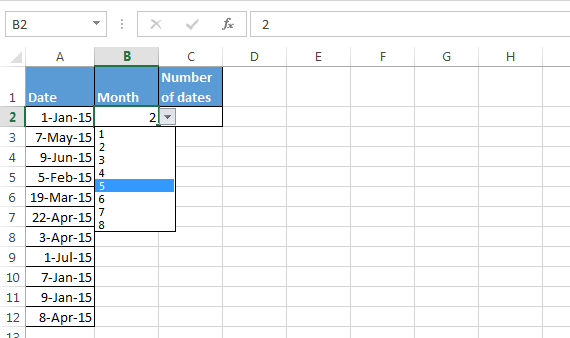• Enter the formula in cell C2
• =SUM(IF(MONTH(A2:A12)=B2,1))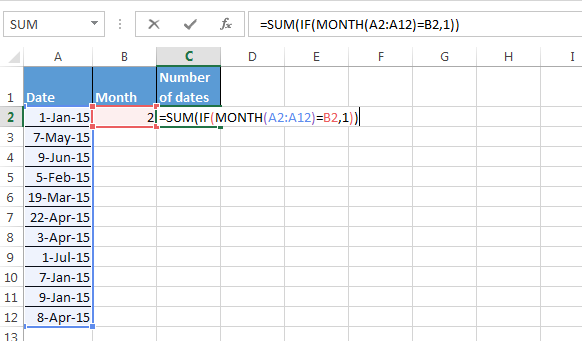• Press the key Ctrl+Shift+Enter
• The function will return 1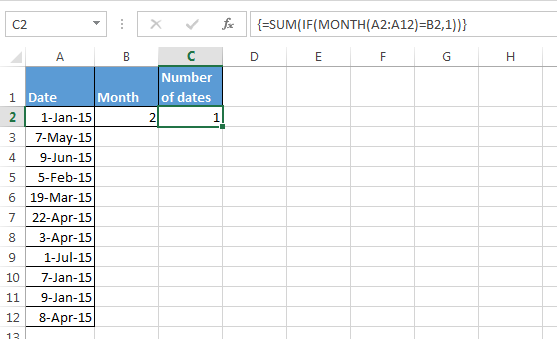How to use Sumproduct function to count the number of dates with the matching month criteria?

• Enter the formula in cell D2
• =SUMPRODUCT(--(MONTH(A2:A12)=B2))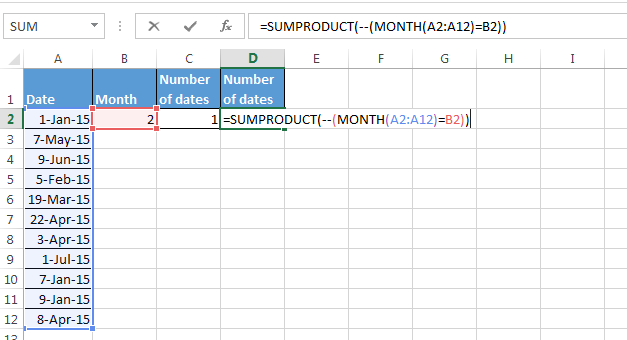• Press Enter.
• The function will return 1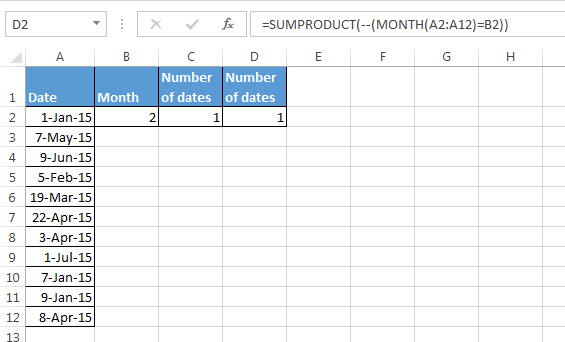Conclusion:-When we select any month from the list, number of dates will get changed, as per selection.We would love to hear from you, do let us know how we can improve, complement or innovate our work and make it better for you. Write us at info@exceltip.com

1.What if there is many blank rows. it shows wrong count for January month.

2.I've tried both the SumProduct, SumIf and CountIf and the SumProduct works for every month BUT January. I've tried multiple different solutions and none count properly. Any suggestions?

3.In a list of dates how do I count how many entries there are for each month?

•Hi ken,

Use this formula {=count(IF(MONTH(A2:A6)=B2,1))}

Terms and Conditions of use

The applications/code on this site are distributed as is and without warranties or liability. In no event shall the owner of the copyrights, or the authors of the applications/code be liable for any loss of profit, any problems or any damage resulting from the use or evaluation of the applications/code.